# Methods Library¶

For convenience, mrsimulator offers the following pre-built methods -

An example of the syntax that all library methods follows is shown below.

from mrsimulator.method import SpectralDimension
from mrsimulator.method.lib import BlochDecaySpectrum

lib_method = BlochDecaySpectrum(
channels=["29Si"],  # list of isotopes
magnetic_flux_density=4.7,  # T
rotor_angle=57.735 * 3.1415 / 180,  # rad
rotor_frequency=10000,  # Hz
spectral_dimensions=[
SpectralDimension(count=512, spectral_width=5e4, reference_offset=10),
],
)


where BlochDecaySpectrum can be replaced with another library method class. Each method has the channels attribute, which is a list of isotopes probed by the method as well as the magnetic_flux_density, rotor_angle, and rotor_frequency attributes which define the global experiment parameters. See Table 6 for more details.

The method object also has the spectral_dimensions attribute, which contains a list of SpectralDimension objects defining the spectral grid. A 2D method will have two spectral dimensions in this list, whereas a 1D method will only have one. See Table 7 for the attributes of a SpectralDimension object.

## Bloch Decay Spectrum¶

The BlochDecaySpectrum class simulates the Bloch decay spectrum.

from mrsimulator.method.lib import BlochDecaySpectrum
from mrsimulator.method import SpectralDimension

method = BlochDecaySpectrum(
channels=["1H"],
rotor_frequency=12500,  # in Hz
rotor_angle=54.735 * 3.14159 / 180,  # in rad
magnetic_flux_density=9.4,  # in tesla
spectral_dimensions=[
SpectralDimension(
count=1024,
spectral_width=25e3,  # in Hz
reference_offset=-4e3,  # in Hz
)
],
)


Examples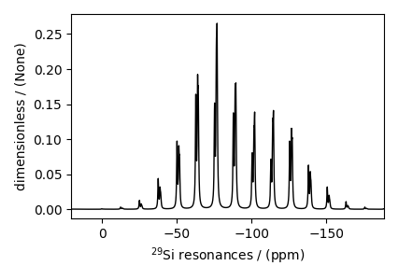Wollastonite, ²⁹Si (I=1/2)

Wollastonite, ²⁹Si (I=1/2)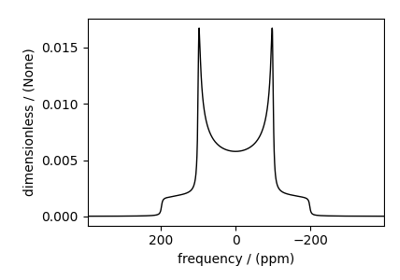Coupled spin-1/2 (Static dipolar spectrum)

Coupled spin-1/2 (Static dipolar spectrum)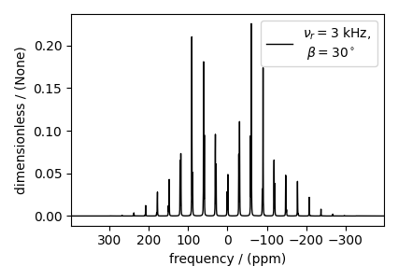Coupled spin-1/2 (CSA + heteronuclear dipolar + J-couplings)

Coupled spin-1/2 (CSA + heteronuclear dipolar + J-couplings)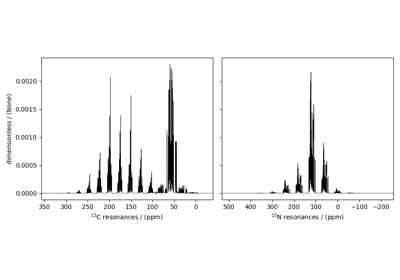Protein GB1, ¹³C and ¹⁵N (I=1/2)

Protein GB1, ¹³C and ¹⁵N (I=1/2)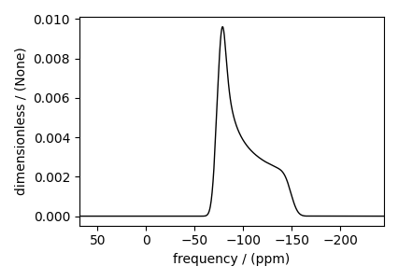Amorphous material, ²⁹Si (I=1/2)

Amorphous material, ²⁹Si (I=1/2)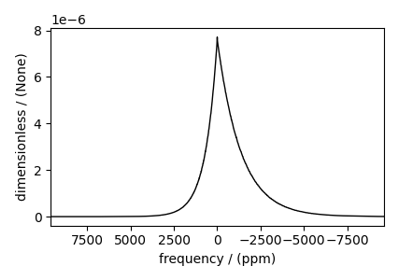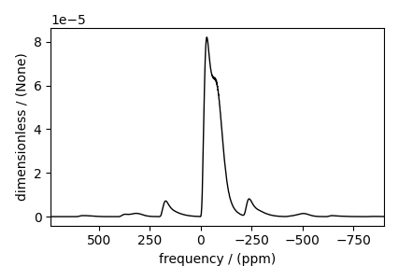Extended Czjzek distribution (Shielding and Quadrupolar)

Extended Czjzek distribution (Shielding and Quadrupolar)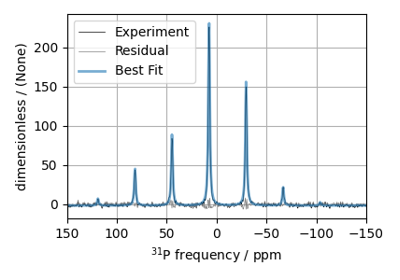³¹P MAS NMR of crystalline Na2PO4 (CSA)

³¹P MAS NMR of crystalline Na2PO4 (CSA)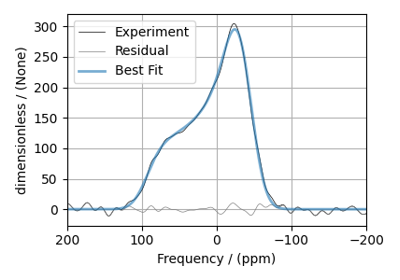³¹P static NMR of crystalline Na2PO4 (CSA)

³¹P static NMR of crystalline Na2PO4 (CSA)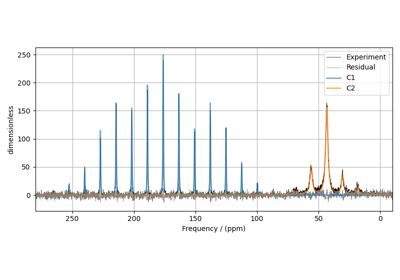¹³C MAS NMR of Glycine (CSA) [960 Hz]

¹³C MAS NMR of Glycine (CSA) [960 Hz]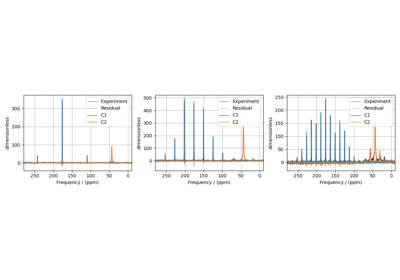¹³C MAS NMR of Glycine (CSA) multi-spectra fit

¹³C MAS NMR of Glycine (CSA) multi-spectra fit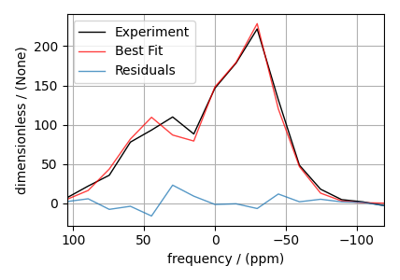1D PASS/MAT sideband order cross-section

1D PASS/MAT sideband order cross-section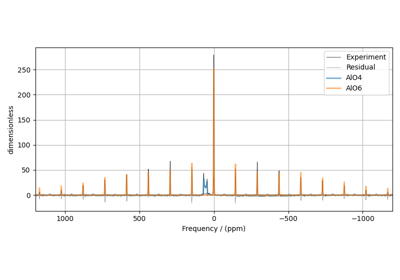²⁷Al MAS NMR of YAG (1st and 2nd order Quad)

²⁷Al MAS NMR of YAG (1st and 2nd order Quad)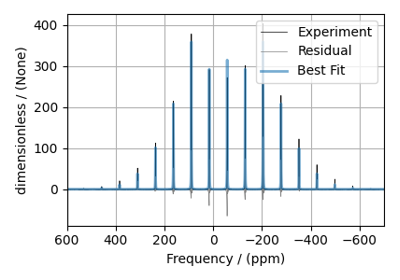²H MAS NMR of Methionine

²H MAS NMR of Methionine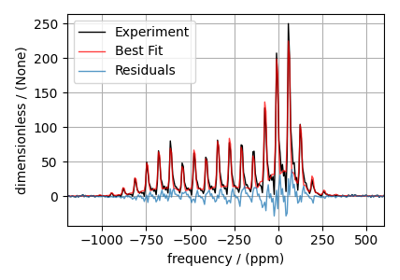¹¹⁹Sn MAS NMR of SnO

¹¹⁹Sn MAS NMR of SnO

## Bloch Decay Central Transition¶

The BlochDecayCTSpectrum class simulates the Bloch decay central transition selective spectrum.

from mrsimulator.method.lib import BlochDecayCTSpectrum
from mrsimulator.method import SpectralDimension

method = BlochDecayCTSpectrum(
channels=["87Rb"],
rotor_frequency=12500,  # in Hz
rotor_angle=54.735 * 3.14159 / 180,  # in rad
magnetic_flux_density=9.4,  # in tesla
spectral_dimensions=[
SpectralDimension(
count=1024,
spectral_width=25e3,  # in Hz
reference_offset=-4e3,  # in Hz
)
],
)


Examples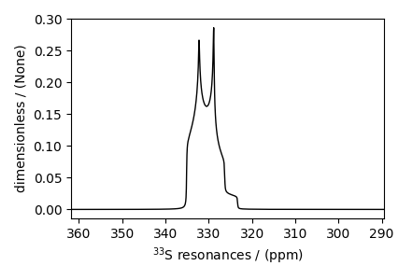Potassium Sulfate, ³³S (I=3/2)

Potassium Sulfate, ³³S (I=3/2)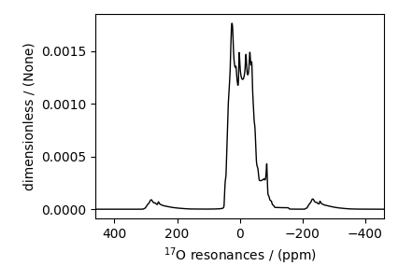Coesite, ¹⁷O (I=5/2)

Coesite, ¹⁷O (I=5/2)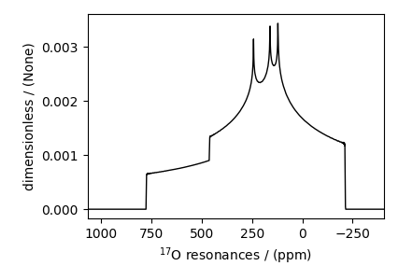Non-coincidental Quad and CSA, ¹⁷O (I=5/2)

Non-coincidental Quad and CSA, ¹⁷O (I=5/2)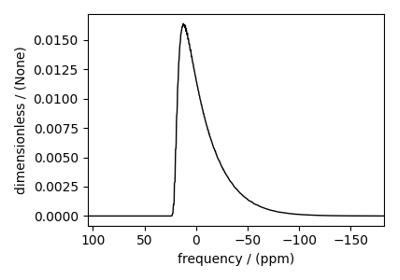Amorphous material, ²⁷Al (I=5/2)

Amorphous material, ²⁷Al (I=5/2)Extended Czjzek distribution (Shielding and Quadrupolar)

Extended Czjzek distribution (Shielding and Quadrupolar)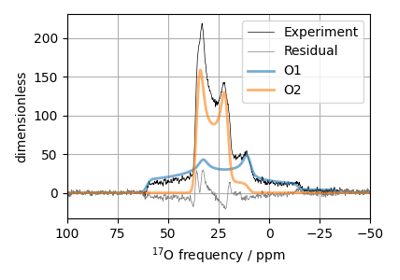¹⁷O MAS NMR of crystalline Na₂SiO₃ (2nd order quad)

¹⁷O MAS NMR of crystalline Na₂SiO₃ (2nd order quad)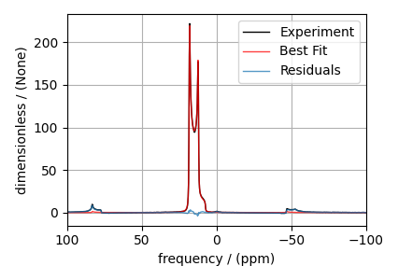¹¹B MAS NMR of Lithium orthoborate crystal

¹¹B MAS NMR of Lithium orthoborate crystal

## Multi-Quantum VAS¶

The ThreeQ_VAS, FiveQ_VAS, and SevenQ_VAS classes all simulate a multiple quantum VAS spectrum. The spinning speed for all three methods is fixed at infinite speed. The spectrum is also sheared such that the correlated dimensions are the isotropic dimension and the VAS dimension.

from mrsimulator.method.lib import ThreeQ_VAS
from mrsimulator.method import SpectralDimension

method = ThreeQ_VAS(
channels=["87Rb"],
magnetic_flux_density=7,  # in T
spectral_dimensions=[
SpectralDimension(
count=128,
spectral_width=3e3,  # in Hz
reference_offset=-2e3,  # in Hz
label="Isotropic dimension",
),
SpectralDimension(
count=512,
spectral_width=1e4,  # in Hz
reference_offset=-5e3,  # in Hz
label="MAS dimension",
),
],
)


The other methods, employing five and seven quantum transitions, can be imported as follows:

from mrsimulator.method.lib import FiveQ_VAS
from mrsimulator.method.lib import SevenQ_VAS


Examples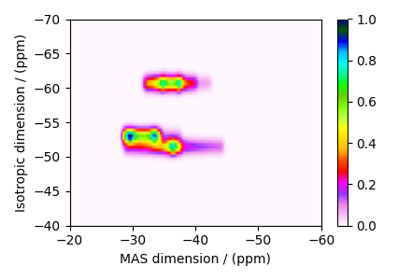RbNO₃, ⁸⁷Rb (I=3/2) 3QMAS

RbNO₃, ⁸⁷Rb (I=3/2) 3QMAS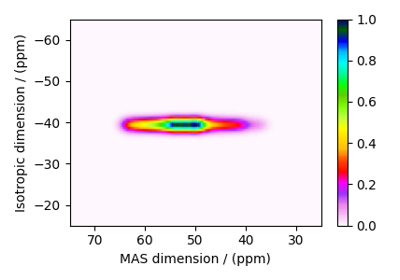Albite, ²⁷Al (I=5/2) 3QMAS

Albite, ²⁷Al (I=5/2) 3QMAS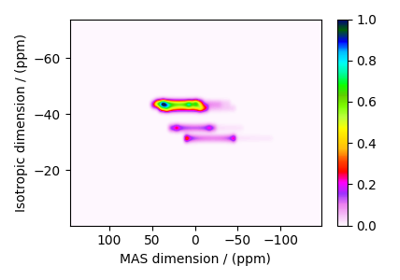Coesite, ¹⁷O (I=5/2) 3QMAS

Coesite, ¹⁷O (I=5/2) 3QMAS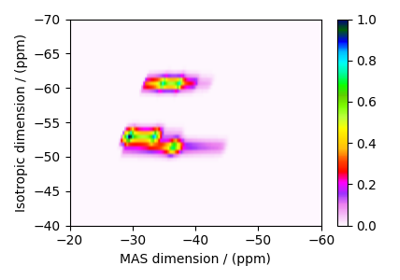Simulating site disorder (crystalline)

Simulating site disorder (crystalline)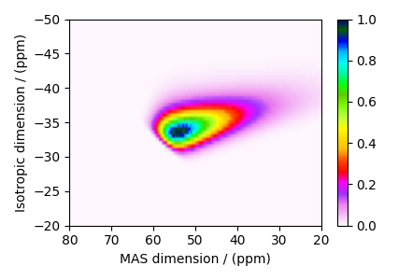Czjzek distribution, ²⁷Al (I=5/2) 3QMAS

Czjzek distribution, ²⁷Al (I=5/2) 3QMAS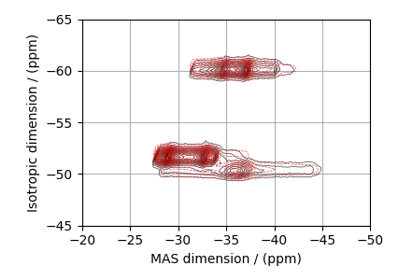⁸⁷Rb 2D 3QMAS NMR of RbNO₃

⁸⁷Rb 2D 3QMAS NMR of RbNO₃

## Satellite-Transition VAS¶

The ST1_VAS and ST2_VAS classes simulate a sheared and scaled satellite and central transition correlation spectrum. The spinning speed for these methods is fixed at infinite speed.

from mrsimulator.method.lib import ST1_VAS
from mrsimulator.method import SpectralDimension

method = ST1_VAS(
channels=["87Rb"],
magnetic_flux_density=9.4,  # in T
spectral_dimensions=[
SpectralDimension(
count=128,
spectral_width=1e3,  # in Hz
reference_offset=-5e3,  # in Hz
label="Isotropic dimension",
),
SpectralDimension(
count=256,
spectral_width=1e4,  # in Hz
reference_offset=-3e3,  # in Hz
label="MAS dimension",
),
],
)


Examples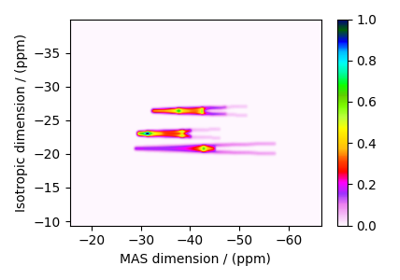RbNO₃, ⁸⁷Rb (I=3/2) STMAS

RbNO₃, ⁸⁷Rb (I=3/2) STMAS

## SSB2D¶

The SSB2D class simulates a sheared 2D finite to infinite speed MAS correlation spectrum. The spinning speed for the second spectral dimension is fixed at infinite spinning speed

from mrsimulator.method.lib import SSB2D
from mrsimulator.method import SpectralDimension

method = SSB2D(
channels=["13C"],
magnetic_flux_density=7,  # in T
rotor_frequency=1500,  # in Hz
spectral_dimensions=[
SpectralDimension(
count=16,
spectral_width=16 * 1500,  # in Hz (= count * rotor_frequency)
reference_offset=-5e3,  # in Hz
label="Sideband dimension",
),
SpectralDimension(
count=512,
spectral_width=1e4,  # in Hz
reference_offset=-4e3,  # in Hz
label="Isotropic dimension",
),
],
)


Examples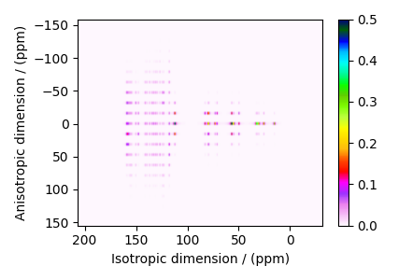Itraconazole, ¹³C (I=1/2) PASS

Itraconazole, ¹³C (I=1/2) PASS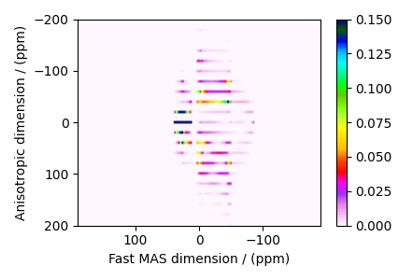Rb₂SO₄, ⁸⁷Rb (I=3/2) QMAT

Rb₂SO₄, ⁸⁷Rb (I=3/2) QMAT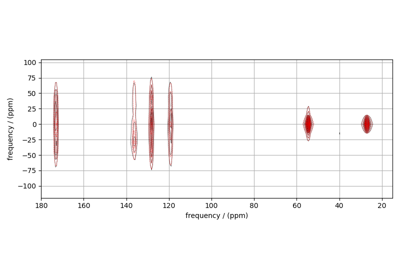¹³C 2D MAT NMR of L-Histidine

¹³C 2D MAT NMR of L-Histidine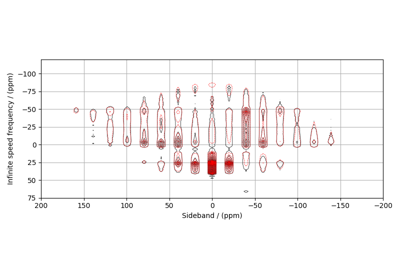⁸⁷Rb 2D QMAT NMR of Rb₂SO₄

⁸⁷Rb 2D QMAT NMR of Rb₂SO₄

## Attribute Summaries¶

Table 6 Attribute description for generic library methods.

Attribute Name

Type

Description

channels

list

A required list of isotopes given as strings over which the given method applies. For example, ["1H"].

magnetic_flux_density

float

An optional float describing the macroscopic magnetic flux density of the applied external magnetic field in tesla. For example, 18.8 tesla. The default value is 9.4 tesla.

rotor_frequency

float

An optional float describing the sample rotation frequency in Hz. For example, 2000 Hz. The default value is 0 Hz.

rotor_angle

float

An optional float describing the angle between the sample rotation axis and the external magnetic field in radians. The default value is the magic angle, 54.735 * 3.14159 / 180 = 0.955305 radians.

spectral_dimensions

list

A list of SpectralDimension objects describing the spectral dimensions for the method.

simulation

CSDM object

A CSDM object representing the spectrum simulated by the method. By default, the value is None. A value is assigned to this attribute when you run the simulation using the run() method.

experiment

CSDM object

An optional CSDM object holding an experimental measurement of the method. The default value is None

Table 7 Spectral dimension attributes for use with library methods.

Attribute Name

Type

Description

count

int

An optional integer representing the number of points, $$N$$, along the spectroscopic dimension. For example, 4096. The default value is 1024.

spectral_width

float

An optional float representing the width, $$\Delta x$$, of the spectroscopic dimension in Hz. For example, 10e3 for 10 kHz. The default value is 25000 Hz.

reference_offset

float

An optional float representing the reference offset, $$x_0$$, of the spectroscopic dimension in Hz. For example, -8000 Hz. The default value is 0.

origin_offset

float

An optional float representing the origin offset, or Larmor frequency, along the spectroscopic dimension in units of Hz. The default value is None and the origin offset is set to the Larmor frequency of isotope from the channels attribute of the method containing the spectral dimension.